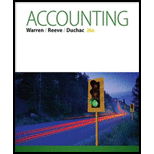# Accounting equation Annie Rasmussen is the owner and operator of Go44, a motivational consulting business. At the end of its accounting period, December 31, 2015, Go44 has assets of $720,000 and liabilities of$180,000. Using the accounting equation and considering each case independently, determine the following amounts: a. Annie Rasmussen, capital, as of December 31, 2015. b. Annie Rasmussen, capital, as of December 31, 2016, assuming that assets increased by $96,500 and liabilities increased by$30,000 during 2016. c. Annie Rasmussen, capital, as of December 31, 2016, assuming that assets decreased by $168,000 and liabilities increased by$15,000 during 2016. d. Annie Rasmussen, capital, as of December 31, 2016, assuming that assets increased by $175,000 and liabilities decreased by$18,000 during 2016. e. Net income (or net loss) during 2016, assuming that as of December 31, 2016, assets were $880,000, liabilities were$220,000, and there were no additional investments or withdrawals.### Accounting (Text Only)

26th Edition
Carl Warren + 2 others
Publisher: Cengage Learning
ISBN: 9781285743615### Accounting (Text Only)

26th Edition
Carl Warren + 2 others
Publisher: Cengage Learning
ISBN: 9781285743615

#### Solutions

Chapter
Section
Chapter 1, Problem 1.7EX
Textbook Problem

## Expert Solution

### Want to see the full answer?

Check out a sample textbook solution.See solution

### Want to see this answer and more?

Experts are waiting 24/7 to provide step-by-step solutions in as fast as 30 minutes!*

See Solution

*Response times vary by subject and question complexity. Median response time is 34 minutes and may be longer for new subjects.